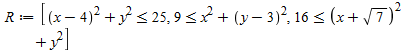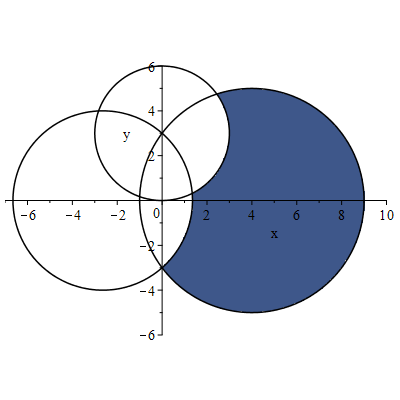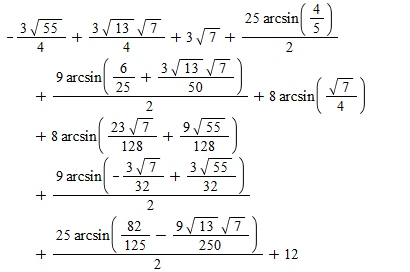## 11977 Reputation

7 years, 254 days

## Bug corrected in inttrans[hilbert]...

Maple

There is a bug in inttrans:-hilbert:

 > restart;
 > inttrans:-hilbert(sin(a)*sin(t+b), t, s); # should be: sin(a)*cos(s+b);   expand(%);(1)
 > ########## correction ##############
 > `inttrans/expandc` := proc(expr, t) local xpr, j, econst, op1, op2;       xpr := expr;             for j in indets(xpr,specfunc(`+`,exp)) do           econst := select(type,op(j),('freeof')(t));           if 0 < nops(econst) and econst <> 0 then               xpr := subs(j = ('exp')(econst)*combine(j/('exp')(econst),exp),xpr)           end if       end do;       for j in indets(xpr,{('cos')(linear(t)), ('sin')(linear(t))}) do           if type(op(j),`+`) then               op1:=select(has, op(j),t); ##               op2:=op(j)-op1;            ##               #op1 := op(1,op(j));               #op2 := op(2,op(j));               if op(0,j) = sin then                   xpr := subs(j = cos(op2)*sin(op1)+sin(op2)*cos(op1),xpr)               else                   xpr := subs(j = cos(op1)*cos(op2)-sin(op1)*sin(op2),xpr)               end if           end if       end do;       return xpr end proc:
 > #######################################
 > inttrans:-hilbert(sin(a)*sin(t+b), t, s); expand(%);(2)
 >

## Multiple integrals over sets defined by ...

Maple 2017

I have just posted an article with this title at Maplesoft Application Center here.
It was motivated by a question posed by  Markiyan Hirnyk  here and a test problem proposed there by Kitonum.

Now I just want to give the promissed complete solution to Kitonum's test:

Compute the plane area of the region defined by the inequalities:

R := [ (x-4)^2+y^2 <= 25, x^2+(y-3)^2 >= 9, (x+sqrt(7))^2+y^2 >= 16 ];plots:-inequal(R, x=-7..10, y=-6..6, scaling=constrained);The used procedures (for details see the mentioned article):

```ranges:=proc(simpledom::list(relation), X::list(name))
local rez:=NULL, r,z,k,r1,r2;
if nops(simpledom)<>2*nops(X) then error "Domain not simple!" fi;
for k to nops(X) do    r1,r2:=simpledom[2*k-1..2*k][]; z:=X[k];
if   rhs(r1)=z and lhs(r2)=z then rez:=z=lhs(r1)..rhs(r2),rez; #a<z,z<b
elif lhs(r1)=z and rhs(r2)=z then rez:=z=lhs(r2)..rhs(r1),rez  #z<b,a<z
else error "Strange order in a simple domain" fi
od;
rez
end proc:

MultiIntPoly:=proc(f, rels::list(relation(ratpoly)), X::list(name))
local r,rez,sol,irr,wirr, rels1, w;
irr:=[indets(rels,{function,realcons^realcons})[]];
wirr:=[seq(w[i],i=1..nops(irr))];
rels1:=eval(rels, irr=~wirr);
sol:=SolveTools:-SemiAlgebraic(rels1,X,parameters=wirr):
sol:=remove(hastype, eval(sol,wirr=~irr), `=`);
end proc:

MeasApp:=proc(rel::{set,list}(relation), Q::list(name='range'(realcons)), N::posint)
local r, n:=0, X, t, frel:=evalf(rel)[];
if indets(rel,name) <> indets(Q,name)  then error "Non matching variables" fi;
r:=[seq(rand(evalf(rhs(t))), t=Q)];
X:=[seq(lhs(t),t=Q)];
to N do
if evalb(eval(`and`(frel), X=~r())) then n:=n+1 fi;
od;
evalf( n/N*mul((rhs-lhs)(rhs(t)),t=Q) );
end proc:
```

Problem's solution:

MultiIntPoly(1, R, [x,y]):  # Unfortunately it's slow; patience needed!evalf(%) = MeasApp(R, [x=-7..10,y=-6..6], 10000); # A rough numerical check
61.16217534 = 59.91480000

## Riemann hypothesis is false! (simple pro...

Maple

# Riemann hypothesis is false! (simple proof)

restart;
assume( s>0, s<1/2, t>0 );
coulditbe(abs(Zeta(s+I*t))=0);

true

# Q.E.D.

Unfortunately coulditbe(Zeta(s+I*t)=0) returns FAIL, but our assertion is already demonstrated!

The moral: the assume facility deserves a much more careful implementation.

## A geometric construction for the Summer ...

Maple

A geometric construction for the Summer Holiday

Does every plane simple closed curve contain all four vertices of some square?

This is an old classical conjecture. See:
https://en.wikipedia.org/wiki/Inscribed_square_problem

Maybe someone finds a counterexample (for non-analytic curves) using the next procedure and becomes famous!

 > SQ:=proc(X::procedure, Y::procedure, rng::range(realcons), r:=0.49) local t1:=lhs(rng), t2:=rhs(rng), a,b,c,d,s; s:=fsolve({ X(a)+X(c) = X(b)+X(d),             Y(a)+Y(c) = Y(b)+Y(d),             (X(a)-X(c))^2+(Y(a)-Y(c))^2 = (X(b)-X(d))^2+(Y(b)-Y(d))^2,             (X(a)-X(c))*(X(b)-X(d)) + (Y(a)-Y(c))*(Y(b)-Y(d)) = 0},           {a=t1..t1+r*(t2-t1),b=rng,c=rng,d=t2-r*(t2-t1)..t2});  #lprint(s); if type(s,set) then s:=rhs~(s)[];[s,s] else WARNING("No solution found"); {} fi; end:

Example

 > X := t->(10-sin(7*t)*exp(-t))*cos(t); Y := t->(10+sin(6*t))*sin(t); rng := 0..2*Pi;(1)
 > s:=SQ(X, Y, rng): plots:-display(    plot([X,Y,rng], scaling=constrained),    plot([seq( eval([X(t),Y(t)],t=u),u=s)], color=blue, thickness=2));## A bug/typo with correct result!...

The help page for  ?procedure has the following example.

 > addList := proc(a::list,b::integer)::integer;     local x,i,s;     description "add a list of numbers and multiply by a constant";     x:=b;     s:=0;     for i in a do         s:=s+a[i];     end do;     s:=s*x: end proc:(1)